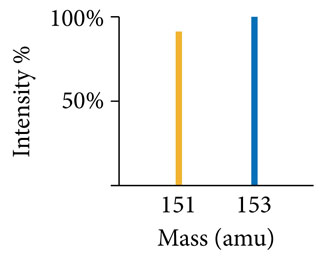# Problem: Use the mass spectrum of europium to determine the atomic mass of europium.Where the peak representing Eu-151 has an exact mass of 150.91986 amu and percent intensity is assumed be to exactly 91.610%, and the peak representing Eu-153 has an exact mass of 152.92138 amu and percent intensity of 100.00%.

###### FREE Expert Solution

First, we have to convert this mass spect data into percent abundance.

The data sets Eu-153 at 100% intensity meaning the percentage that we see for Eu-151 is relative to Eu-153.

To get percent abundance we have to make it relative to the total. We do that with the equations below:

81% (7 ratings)###### Problem Details

Use the mass spectrum of europium to determine the atomic mass of europium.Where the peak representing Eu-151 has an exact mass of 150.91986 amu and percent intensity is assumed be to exactly 91.610%, and the peak representing Eu-153 has an exact mass of 152.92138 amu and percent intensity of 100.00%.